# Characterization of digital medical images utilizing support vector machines

## Abstract

### Background

In this paper we discuss an efficient methodology for the image analysis and characterization of digital images containing skin lesions using Support Vector Machines and present the results of a preliminary study.

### Methods

The methodology is based on the support vector machines algorithm for data classification and it has been applied to the problem of the recognition of malignant melanoma versus dysplastic naevus. Border and colour based features were extracted from digital images of skin lesions acquired under reproducible conditions, using basic image processing techniques. Two alternative classification methods, the statistical discriminant analysis and the application of neural networks were also applied to the same problem and the results are compared.

### Results

The SVM (Support Vector Machines) algorithm performed quite well achieving 94.1% correct classification, which is better than the performance of the other two classification methodologies. The method of discriminant analysis classified correctly 88% of cases (71% of Malignant Melanoma and 100% of Dysplastic Naevi), while the neural networks performed approximately the same.

### Conclusion

The use of a computer-based system, like the one described in this paper, is intended to avoid human subjectivity and to perform specific tasks according to a number of criteria. However the presence of an expert dermatologist is considered necessary for the overall visual assessment of the skin lesion and the final diagnosis.

## Background

So far, dermatologists have based the diagnosis of skin lesions on the visual assessment of pathological skin and the evaluation of macroscopic features. Therefore the diagnosis has been highly dependent on the observer's experience and on his or her visual acuity. However, the human vision lacks accuracy, reproducibility and quantification in gathering information from an image; thus systems that are able to evaluate images in an objective manner are obviously needed [1, 2].

Recently there has been a significant increase in the level of interest in image morphology, full-color image processing, image recognition, and knowledge – based image analysis systems for skin lesions. The quantification of tissue lesion features in digital images has been proven to be of essential importance in clinical practice . Several tissue lesions can be identified through measurable features that are extracted by digital images [4, 5]; in addition the use of digital image features may help in an objective follow up study of skin lesion progression and test the efficacy of therapeutic procedures .

The objective of this paper is to present an efficient methodology for the characterization of dermatological images based on measurements of extracted image features using the support vector machine (SVM) algorithm. The methodology has been applied for the recognition of melanoma versus dysplastic naevus. Other classification methods, such as discriminant analysis and neural networks, were used for the same problem and the results were compared with the SVM algorithm performance .

## Methods

### Image acquisition and feature extraction

A significant issue, considered decisive for the efficiency of image analysis based characterization is the reproducibility of the captured images. In our research, for the image acquisition, we have used a prototype described in . The specific system includes a standardized illumination and capturing geometry with polarizing filters and a series of software corrections: Calibration to Black, White and Color for color constancy, Internal camera Parameters adjustment and Pose extraction for stereo vision, Shading correction and Noise Filtering for color quality. The validity of the calibration procedure and the images' reproducibility were tested by capturing sample images in three different lighting conditions: dark, medium and intense lighting. For each case the average values of the three color planes RGB and their standard deviations were calculated; the measured error differences ranged between 0,7 and 12,9 (in the 0–255 scale). Preliminary experiments for stereo measurements provided repeatability of about 0.3 mm.

The analysis of dermatological digital images is performed by measurements on the pixels that represent a segmented object, thus the skin lesion. The measured pixels allow non-visible to human perception, features to be computed. The segmentation of the skin image could be accomplished either automatically by unsupervised segmentation algorithms [12, 13], or with the help of an expert physician. In our research we asked from an expert dermatologist to manually determine the lesion border. In automated diagnosis of skin lesions, feature design is based on the so-called ABCD-rule of dermatology. ABCD represent the Asymmetry, Border structure, variegated Color, and the Diameter of the lesion and define the basis for a diagnosis by a dermatologist . Thus, two feature categories were calculated: the border based features, which are limited on computations regarding the lesion border and the color based features, which refer to measurements in pixels inside the lesion border [15, 16].

More specifically, the computed border-based features were the Area of the lesion, the Border Irregularity, the Border Thinness Ratio, and the Border Asymmetry. The acquired color features were based on measurements on the RGB color plane and other color planes such as the HIS (Hue, Intensity, Saturation), and the LAB plane, corresponding to Spherical Coordinates. Color variegation was also calculated by measuring standard deviations of the RGB channels and chromatic differences in the CIE color plane inside the border. Finally a heuristic linear transformation presented in  and  was also incorporated.

The basic aim was to construct a classification system for skin lesions, enabling the distinction of malignant melanoma from dysplastic naevus. Two groups of data will be considered. The first group (denoted MEL) consists of cases of malignant melanoma, with measurements taken on the entire extent of the lesion. The second group (denoted DSP) comprises cases of dysplastic naevus. Figures 1 and 2 depicts typical examples of a malignant melanoma (Figure 1) and a naevus (Figure 2). Detailed analysis was carried out considering the classification of MEL versus DSP cases.

### Classification methods

#### Support vector machines

The Support Vector Machines (SVMs) is a novel algorithm for data classification and regression. They were introduced by Vapnic in 1995 and are clearly connected with the statistical learning theory . The SVM is an estimation algorithm that separates data in two classes, but since all classification problems can be restricted to consideration of the two-class classification problem without loss of generality, SVMs can be applied in classification problems in general. SVMs allow the expansion of the information provided by a training data set as a linear combination of a subset of the data in the training set (support vectors). These vectors locate a hypersurface that separates the input data with a very good degree of generalization. The SVM algorithm is a learning machine; therefore it is based on training, testing and performance evaluation, which are common steps in every learning procedure. Training involves optimization of a convex cost function where there are no local minima to complicate the learning process. Testing is based on the model evaluation using the support vectors to classify a test data set. Performance is based on error rate determination as test set data size tends to infinity.

Consider the case of:

• a set of N training data points {(X 1, y1),...,(X N, yN)}

• a hyperplane

H: y = w·X-b = 0     (1)

where w is normal to the hyperplane, b/||w|| the perpendicular distance to the origin and ||w|| the Euclidean norm of w

• two hyperplanes parallel to H

H1: y = w·X-b = +1     (3)

H2: y = w·X-b = -1     (4)

with the conditions that there are no data points between H1 and H2

The above situation is illustrated in Figure 3. If d+ (d-) is the shortest distance from the separating hyperplane H to the closest positive (negative) data point where the hyperplanes H1 (H2) is located, then the distance between the hyperplanes H1 and H2 is d+ + d-. Since d+ = d- = 1/||w||, then the margin equals 2/||w||. The problem is to find the pair of hyperplanes that give the maximum margin: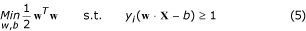The parameters w, b control the function and are called weight vector and bias respectively. The optimization problem presented in equation (5) can be stated in a convex, quadratic problem in (w, b) in a convex set. Using the Lagrangian formulation, the constraints will be replaced by constraints on the Lagrange multipliers themselves. Additionally in this reformulation, as a consequence the training data will only appear in the form of dot product between data vectors. Introducing Lagrangian multipliers α1,...,αN ≥ 0, a Lagrangian function for the optimization problem can be defined: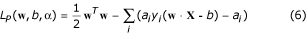Using the Wolfe dual formulation and the constraints of the Lagrangian optimization problem [19, 20], the parameters αi can be calculated and the parameters w, b which specify the separating hyperplane can be calculated using the following equations: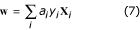a i (y i (w ·X i + b) - 1) = 0 i     (8)

According to equation (7), the parameters αi that are not equal to zero correspond to data X i, yi that are the support vectors (Figure 3).

If the surface separating the two classes is not linear, the data points can be transformed to another high dimensional feature space where the problem is linearly separable. If the transformation to the high dimensional space is Φ() then the Lagrangian function can be expressed as: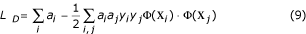The dot product Φ(X i)Φ(X j) in that high dimensional space defines a kernel function k(X i, X j) and therefore it is not necessary to be explicit about the transformation Φ() as long as it is known that the kernel function corresponds to a dot product in some high dimensional feature space . This case is presented in Figure 4.

With a suitable kernel, SVM can separate in the feature space the data that in the original input space was non-separable. There are many kernel functions that can be used, for example: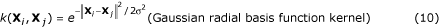k(X i ,X j ) = (X i ·X j + m)p (the polynomial kernel)     (11)

A kernel function has a good performance if the support vectors that are calculated by using the corresponding transformation are few and the classification of the test data is successful.

To sum up, in order to separate a data set, a train data set (X, Y) is selected, the optimization problem is solved and the parameters αi, w, b are calculated. Then, a given data vector X of the initial data set is classified according to the value of sgn(w·X*+b). The performance of the support vectors calculated is tested using a test data set derived from the initial data set.

#### Discriminant analysis

The main aim of discriminant analysis [23, 24] is to allocate an individual to one of two or more known groups, based on the values of certain measurements x. The discriminant procedure identifies that combination (in the commonest case, as applied here, the linear combination) of these predictor variables that best characterizes the differences between the groups. The procedure estimates the coefficients, and the resulting discriminant function can be used to classify cases. The analysis can also be used to determine which elements of the vector of measurements x are most useful for discriminating between groups. This is usually done by implementing stepwise algorithms, as in multiple regression analysis, either by successively eliminating those predictor variables that do not contribute significantly to the discrimination between groups, or by successively identifying the predictor variables that do contribute significantly.

One important discriminant rule is based on the likelihood function. Consider k populations or groups Π1,...,Πk, k ≥ 2 and suppose that if an individual comes from population Πj, it has probability density function fj(x). The rule is to allocate x to the population Πj giving the largest likelihood to x

Lj(x) = max Li(x)     (12)

In practice, the sample maximum likelihood allocation rule is used, in which sample estimates are inserted for parameter values in the pdf's fj(x). In a common situation, let these densities be multivariate normal with different means μ i but the same covariance matrix Σ. Unbiased estimates of μ 1 ,...,μ g are the sample means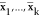, while

S u = Σ ni S i / (n-k)     (13)

is an unbiased estimator of Σ, where S i is the sample covariance matrix of the ith group. In particular when k = 2 the sample maximum likelihood discriminant rule allocates x to Π1 if and only if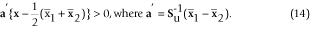Another important approach is Fisher's Linear Discriminant Function. In this method, the linear function a'x is found that maximizes the separation between groups in the sense of maximizing the ratio of the between-groups sum of squares to the within-groups sum of squares,

a'Ba/ a'Wa     (15)

The solution to this problem is the eigenvector of W -1 B that corresponds to the largest eigenvalue. In the important special case of two populations, Fisher's LDF becomes: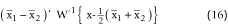The discrimant rule is to allocate a case with values x to Π1 if the value of the LDF is greater than zero and to Π2 otherwise. This allocation rule is exactly the same as the sample ML rule for two groups from the multivariate normal distribution with the same covariance matrix. However, the two approaches are quite different in respect of their assumptions. Whereas the sample ML rule makes an explicit assumption of normality, Fisher's LDF contains no distributional assumption, although its sums of squares criterion is not necessarily a sensible one for all forms of data.

Preliminary data exploration by constructing normal probability plots for each variable, in each group separately indicated that most variables measured in this study followed distributions that were reasonably close to the normal distribution. It was therefore decided to apply discriminant analysis to the data as they stood, and to defer further investigation of possible transformations of variables to a later time when more cases would be available for analysis.

#### Neural networks

The methodology of neural networks involves mapping a large number of inputs into a small number of outputs and it is therefore frequently applied to classification problems in which the predictors x form the inputs and a set of variables denoting group membership represent the outputs [25, 26]. It is thus a major alternative to discriminant analysis and a comparison between the results of these two entirely different approaches is interesting. Neural networks are very flexible as they can handle problems for which little is known about the form of the relationships.

In the basic feed-forward neural network, an input layer sends signals to a hidden middle layer, as in Figure 5. Each neuron in the hidden layer weights the various inputs and sends a signal on to neurons in the final output layer, where again the weighted signals are aggregated to generate final output values. The hidden layers can be thought of as a form of intermediate processing of the data. One hidden layer will normally suffice for classification problems .

Feed-forward neural networks with one hidden layer and allowing skip-layer connections directly from the inputs to the output were fitted in this study using the S-Plus programming language. Because of the excessively large number of input variables available, and the lack of any automated procedure for variable selection, it was decided to reduce the number of inputs to the neural network models by taking principal components of the 20 variables and alternatively by using the variable combinations that had been selected in the corresponding discriminant analyses. Principal components analysis is the commonest method of reducing the dimensionality of multivariate statistical data . However, it uses a variance maximization criterion and this is not necessarily relevant to discrimination. Therefore, other model selection criteria will be applied at a later stage of this work. Various criteria have been developed in conventional statistics for assessing the performance of trained models without the use of validation data. Well known examples include Mallows' Cp statistic and the Akaike information criterion . The general form is:

Prediction error = Training error + Complexity term

in which the complexity term represents a penalty which increases as the number of free parameters in the model grows. The minimum value of the criterion is a trade-off between the increased training error due to fitting too simple a model and the high complexity value due to fitting a complex model. A form suitable for non-linear models is the Generalized Prediction Error criterion :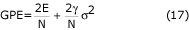where γ is the effective number of parameters in the network, E is the error sum of squares, N is the number of data points in the training set and σ2 is the variance of the noise of the data.

## Results and discussion

In order to apply the support vector methodology for the classification of MEL and DSP data, a train data set of 17 cases was used. Our sample was patients that arrived at the Dept of Plastic Surgery and Dermatology in Athens General Hospital. We have captured images of all melanomas and all suspicious dysplastic naevi, within a period of 6 months. The total number of lesions captured was 17: 7 melanomas and 10 dysplastic naevi. The mean thickness of melanomas lesions was measured during biopsy afterwards at approximately 1.5 mm penetration through the skin. Feature statistics of the analyzed lesions are depicted in Table 1.

For the selection of the kernel function, different polynomial kernel functions were tried on subsets of the 17 cases in order to find the less complex kernel function that results in low number of support vectors comparing to the train set. The results for the kernel functions that were tried are shown in Table 2. In this way, a Gaussian radial base kernel function, which is shown in equation 10, was used; the σ value used was 4 and seven support vectors were calculated using the train set of the 17 cases . The support vectors calculated using the Gaussian radial base kernel function with the σ value equal to 4 were the fewest with 94.1% successful classification of the test data set. The support vectors and the corresponding αi values are presented in Table 3. The bias b was calculated equal to 0. These support vectors were tested using all the cases of malignant melanoma denoted as MEL and dysplastic naevus denoted as DSP and it performed quite well, classifying them with 94.1% successful classification.

In order to evaluate the performance of the SVM algorithm, we have implemented for the same problem the two other previously discussed classification methods. The method of discriminant analysis classified correctly 88% of cases (71% of MEL and 100% of DSP). The neural networks models also performed very well. Using four principal components as input, the success rate achieved was 94.1%. This was reduced to 84.6% correct classification (82% of MEL and 87% of DSP) using only the first two principal components. Using Area and Thinness Ratio for input – that is, the two significant predictors identified – gave 88% correct classification, exactly as in the discriminant analysis. Both methods, discriminant analysis and the neural networks misclassified the same cases of malignant melanoma as dysplastic naevus.

## Conclusions

The technical achievements of recent years in the areas of image acquisition and processing allow the improvement and lower cost of image analysis systems. Such tools may serve as diagnostic adjuncts for medical professionals for the confirmation of a diagnosis, as well as for the training of new dermatologists . The introduction of diagnostic tools based on intelligent decision support systems is also capable of enhancing the quality of medical care, particularly in areas where a specialized dermatologist is not available. The inability of general physicians to provide high quality dermatological services leads them to wrong diagnoses, particularly in evaluating fatal skin diseases such as melanoma. In such cases, an expert system may detect the possibility of a serious skin lesion and warn of the need for early treatment.

In the present paper, the support vector machines algorithm has been implemented to the problem of the recognition of malignant melanoma versus dysplastic naevus. Furthermore the discriminant analysis and the neural networks methodology for data classification have been implemented. The SVM algorithm performed excellently achieving 94.1% correct classification, which is marginally better than the performance of the other two classification methodologies. In general, the SVM algorithm exhibit good generalization performance and training involves optimization of a convex cost function where there are no local optima to complicate the learning process. Although the choice of the kernel is a limitation of the SVM approach, it has been noticed that when different kernel functions are used, they empirically lead to very similar classification accuracy.

It should be noted though that this is a preliminary study and it is now necessary to examine more patients in order to increase the number of cases. This will clarify the issue of selecting the most powerful variables for classification.

The use of a computer-based system, like the one described in this paper is intended to avoid human subjectivity and to perform specific tasks according to a number of criteria. However the presence of an expert dermatologist is considered necessary for the overall visual assessment of the skin lesion and the final diagnosis.

## References

1. Hansen G, Sparrow E, Kokate JY, Leland KJ, Iaizzo PA: Wound status evaluation using color image processing. IEEE Transactions on Medical Imaging. 1997, 16: 78-86. 10.1109/42.552057.

2. Herbin M, Bon F, Venot A, Jeanlouis F, Dubertret ML, Dubertret L, Strauch G: Assessment of healing kinetics through true color image processing. IEEE Transactions on Medical Imaging. 1993, 12: 39-43. 10.1109/42.222664.

3. Hall PN, Claridge E, Smith M: Computer screening for early detection of melanoma – is there a future?. Br J Dermatol. 1995, 132: 325-338.

4. Ganster H, Pinz P, Rohrer R, Wildling E, Binder M, Kittler H: Automated melanoma recognition. IEEE Transactions on Medical Imaging. 2001, 20: 233-239. 10.1109/42.918473.

5. Seidenari S, Burroni M, Dell'Eva G, Pepe P, Belletti B: Computerized evaluation of pigmented skin lesion images recorded by a videomicroscope: Comparison between polarizing mode observation and oil/slide mode observation. Skin Res Technol. 1995, 1: 187-191.

6. Kjoelen M, Thompson M, Umbaugh S, Moss R, Stoecker W: Performance of Artificial Intelligence Methods in Automated Detection of Melanoma. IEEE Engineering Medicine and Biology. 1995, 14: 411-416. 10.1109/51.395323.

7. Maglogiannis I: Automated Segmentation and Registration of Dermatological Images. Proceedings of the 2002 International Conference on Parallel and Distributed Processing Techniques and Applications USA. 2002, 121-126.

8. Nishik M, Foster C: Analysis of Skin Erythema Using True Color Images. IEEE Transactions on Medical Imaging. 1997, 16: 711-716. 10.1109/42.650868.

9. Umbaugh SE, Wei Y, Zuke M: Feature Extraction in Image Analysis. IEEE Engineering in Medicine and Biology. 1997, 16: 62-73. 10.1109/51.603650.

10. Dreiseitl S, Ohno-Machado L, Kittler H, Vinterbo S, Billharrt H, Binder M: A comparison of Machine Learning Methods for the Diagnosis of Pigmented Skin Lesions. Journal of Biomedical Informatics. 2001, 34: 28-36. 10.1006/jbin.2001.1004.

11. Maglogiannis I, Kosmopoulos DI: A system for the acquisition of reproducible digital skin lesion images. Technol Health Care. 2003, 11: 425-441.

12. Grana C, Pellacani G, Cucchiara R, Seidenari S: A New Algorithm for Border Description of Polarized Light Surface Microscopic Images of Pigmented Skin Lesions. IEEE Transactions on Medical Imaging. 2003, 22: 959-964. 10.1109/TMI.2003.815901.

13. Chung DH, Sapiro G: Segmenting skin lesions with partial-differential-equations-based image processing algorithms. IEEE Transactions on Medical Imaging. 2000, 19: 763-767. 10.1109/42.875204.

14. Bränström R, Hedblad MA, Krakau I, Ullén H: Laypersons' perceptual discrimination of pigmented skin lesions. Journal of the American Academy of Dermatology. 2002, 46: 667-673. 10.1067/mjd.2002.120463.

15. Ballard DH, Brown CM: Computer Vision. 1982, Prentice Hall Inc

16. Gonzalez CR, Woods ER: Digital Image Processing. 1995, New York: Addison-Wesley

17. Ohta YI, Kanade T, Sakai T: Color Information for Region Segmentation. Computer Graphics and Image Proccesing. 1980, 13: 222-241.

18. Round AJ, Duller WG, Fish PJ: Color Segmentation For Lesion Clasification. In Proceedings of the 19th IEEE/EMBS USA. 1997, 582-585.

19. Burges C: A tutorial on support vector machines for pattern recognition. [http://www.kernel-machines.org/]

20. Christianini N, Shawe-Taylor J: An introduction to support vector machines. 2000, Cambridge University Press

21. Schölkopf B: Statistical learning and kernel methods. [http://research.Microsoft.com/~bsc]

22. Campbell C: Kernel methods: a survey of current techniques. [http://www.kernel-machines.org/]

23. Mardia KV, Kent JT, Bibby JM: Multivariate Analysis. 1979, London Academic Press

24. Weiss SM, Kulikowski CA: Computer Systems that Learn: Classification and Prediction Methods from Statistics, Neural Nets, Machine Learning and Expert Systems. 1991, San Mateo Morgan Kaufmann

25. Ripley BD: Neural networks and related methods for classification. Journal of the Royal Statistical Society B. 1994, 56: 409-456.

26. Bishop CM: Neural Networks for Pattern Recognition. 1995, Oxford Clarendon Press

27. Moody JE: The effective number of parameters: an analysis of generalization and regularization in nonlinear learning systems. In Advances in Neural Information Processing Systems. Edited by: Moody JE, Hanson SJ and Lippmann RP. 1992, Morgan Kaufmann, 4: 847-854.

28. Rocco CM, Moreno JA: Fast Monte Carlo reliability evaluation using support vector machine. Reliability Engineering and System Safety. 2002, 76: 237-243. 10.1016/S0951-8320(02)00015-7.

29. Kalyanmoy Deb A, Raji R: Reliable classification of two-class cancer data using evolutionary algorithms. Biosystems. Article in Press by Elsevier

30. Maglogiannis I, Caroni C, Pavlopoulos S, Karioti V: Utilizing Artificial Intelligence for the Characterization of Dermatological Images. In Proceedings of the 4th International Conference Neural Networks and Expert Systems in Medicine and Healthcare. 2001, 362-368.

## Acknowledgements

The skin lesion images used for the SVM algorithm efficiency tests were acquired from patients in the General Hospital of Athens G. Gennimatas with the help of the medical personnel of the department of Plastic Surgery and Dermatology.

## Author information

Authors

### Corresponding author

Correspondence to Ilias G Maglogiannis.

None declared.

### Authors' contributions

IM carried out the collection and image processing of the samples, as well as the modeling and implementation of the classification. EZ assisted with the modeling and implementation of the SVM algorithm.

## Authors’ original submitted files for images

Below are the links to the authors’ original submitted files for images.

## Rights and permissions

Reprints and Permissions

Maglogiannis, I.G., Zafiropoulos, E.P. Characterization of digital medical images utilizing support vector machines. BMC Med Inform Decis Mak 4, 4 (2004). https://doi.org/10.1186/1472-6947-4-4

• Accepted:

• Published:

• DOI: https://doi.org/10.1186/1472-6947-4-4

### Keywords

• Support Vector Machine
• Discriminant Analysis
• Kernel Function
• Thinness Ratio
• Support Vector Machine Algorithm